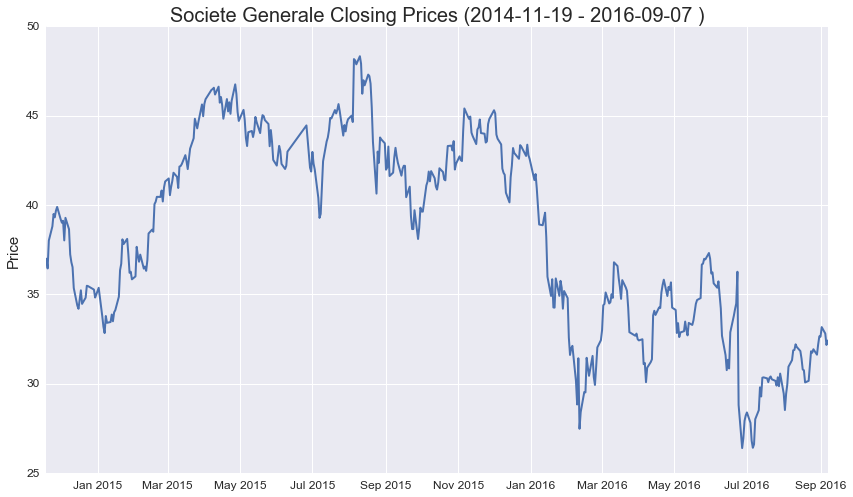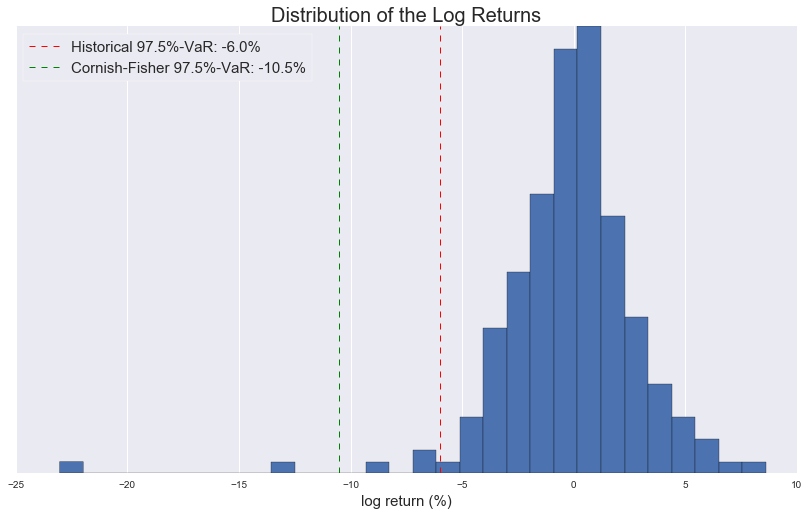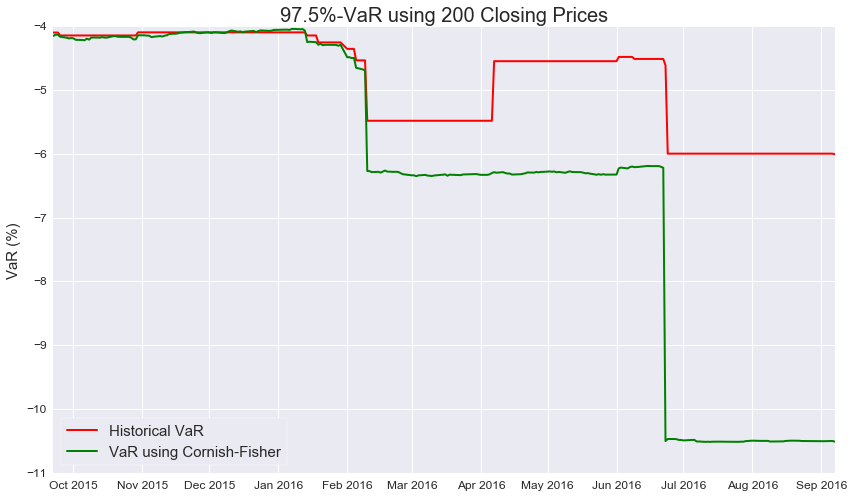### Risk Management Solutions

September 8, 2016

# VaR Equivalent Volatility

### Introduction

Financial products depending on one or more indices or stocks are subject to market fluctuations. The value of the product can change up or down leading to a possible gain or a loss. The European Supervisory Authorities (ESMA, EIOPA and EBA) have made it clear that investors are to be informed in a standardised way on the embedded risk of these products: a new KID was born replacing the KIID. The new proposal had January 1, 2017 as its implementation date. A recent vote in the European Parliament has definitively changed the agenda and a delay is certainly to be expected. In their new proposal regulators have embraced the concept of the Cornish-Fisher expansion in order to allow the transformation of a Value at Risk number (VaR) into a volatility-like measure such as the VaR Equivalent Volatility (VEV). This VEV number gets eventually assigned to a market risk-category (MRM) ranging from a scale of 1 to 7:

MRM
1 < 0.5%
2 0.5% - 5%
3 5.0% - 12%
4 12% - 20%
5 20% -30%
6 30% -80%
7 > 80%

### Moving away from normality

Since the level of the VEV is derived from the VaR, a logical first step is to calculate the value at risk (VaR) for a particular financial instrument. Here the regulators have opted for the $97.5\%$-VaR. The probability of a loss exceeding this amount is $2.5\%$. On a sample of 200 closing prices, the 2.5% VaR level corresponds to the sixth smallest return. A logical starting point when calculating the VaR-number of a particular holding is the assumption that the price returns are normally distributed. One can estimate the mean and variance of the underlying normal distribution using historical price observations. The $2.5\%$-percentile gets us the estimation of the VaR.

The disadvantage of this method is the assumption of normality. Stock price returns are often right or left skewed, which makes the assumption of normality invalid. A solution is to make use of the Cornish-Fisher expansion. The Cornish–Fisher expansion is an asymptotic expansion used to approximate the quantiles of a probability distribution based on its moments. The Cornish-Fisher expansion delivers us a closed-form formula to obtain percentiles for non-normal distributions when limiting ourselves for example to only the first four moments of the distribution: the mean, the variance, the skewness and the excess kurtosis. The Cornish-Fisher expansion hence gives us a method to calculate the VaR directly from these four moments:

We have the following formula to derive the VaR of the price returns at the 97.5% confidence level: \begin{equation}VaR_{0.975}=\sigma(-1.96+0.474s-0.0687k + 0.146s^2)-\frac{1}{2}\sigma^2 \end{equation} with $\sigma$ the standard deviation, $s$ the skewness and $k$ the excess kurtosis.

### Example

Using as illustrative example the daily log returns of FedEx we calculate the daily VaR using the two different methods.
• The historical $97.5\%$-VaR corresponds with the sixth smallest return, which is in this particular example $-3.45\%$. So only in $2.5\%$ of the business days a log return smaller than this number is expected to be observed.
• To obtain the Cornish-Fisher VaR (limiting ourselves to the first four moments), we first estimate the standard deviation, skew and kurtosis. For this example the parameter estimates are $$\begin{equation} \begin{array}{lll} \sigma &= &0.0166\\ s &=& 1.1247\\ k &= &10.4444\\ \end{array} \end{equation}$$
Using the above equation for the Cornish-Fisher VaR, we obtain a $97.5\%$-VaR of $-3.26\%$.
The VaR numbers are different for the two methods, although the differences are small. In this example, the Cornish-Fisher expansion holds. In certain circumstances, the VaR obtained through the first four moments, will not always provide a good approximation. If the skew and kurtosis exceed certain limits, the Cornish-Fisher expansion is not valid. In a later contribution we will handle in detail a couple of numerical examples to illustrate this.### Var Equivalent Volatility

Now that we know how to calculate the VaR, the second step is to obtain the VaR equivalent volatility. We use the Cornish-Fisher expansion, while taking the skew and excess kurtosis equal to zero. This enables us to derive the standard deviation from the VaR. The obtained standard deviation is our VEV and the solution for this daily VEV number is: $$\begin{equation} VEV = \sqrt{3.842-2\times VaR}-1.96. \end{equation}$$ Depending on the VaR we use, we obtain different values for the VEV. In our example where we analysed Societe Generale's share price movements, the VEV corresponding to a VaR of $-6.0\%$ is $3.05\%$ and the VEV corresponding with the Cornish-Fisher VaR of $-10.5\%$ is $5.30\%$.
The annualised VEV numbers are respectively $48.19\%$ and $83.7\%$, using the square root of time rule with 250 trading days.
The advantage of using Cornish-Fisher to obtain the VEV is that we have a closed form formula. But we have to remark that the formula is not always valid. There are limits on the skew and kurtosis of a distribution under which the Cornish-Fisher holds. Very often practitionars also mix up the skewness and kurtosis parameters in the formula with the actual skewness and kurtosis of the distribution.

### VaR Comparison

The figure below illustrates the difference between the VaR obtained using Cornish-Fisher constrainted to the first four moments compared to a historical VaR on the same time series of 200 closing prices.